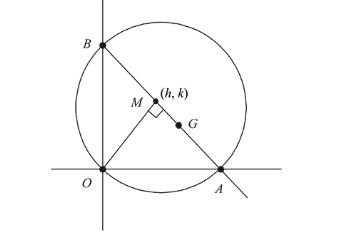# If a circle of radius R passes through the originQuestion:

If a circle of radius $\mathrm{R}$ passes through the origin $\mathrm{O}$ and intersects the coordinate axes at $\mathrm{A}$ and $\mathrm{B}$, then the locus of the foot of perpendicular from $\mathrm{O}$ on $\mathrm{AB}$ is :

1. (1) $\left(x^{2}+y^{2}\right)^{2}=4 R^{2} x^{2} y^{2}$

2. (2) $\left(x^{2}+y^{2}\right)^{3}=4 R^{2} x^{2} y^{2}$

3. (3) $\left(x^{2}+y^{2}\right)^{2}=4 R x^{2} y^{2}$

4. (4) $\left(x^{2}+y^{2}\right)(x+y)=R^{2} x y$

Correct Option: , 2

Solution:

As $\angle A O B=90^{\circ}$Let $A B$ diameter and $M(h, k)$ be foot of perpendicular, then

$M_{A B}=-$

Then, equation of $A B$

$(y-k)=\frac{-h}{k}(x-h)$

$\Rightarrow \quad h x+k y=h^{2}+k^{2}$

Then, $A\left(\frac{h^{2}+k^{2}}{h}, 0\right)$ and $B\left(0, \frac{h^{2}+k^{2}}{k}\right)$

$\because A B$ is the diameter, then

$A B=2 R$

$\Rightarrow A B^{2}=4 R^{2}$

$\Rightarrow \quad\left(\frac{h^{2}+k^{2}}{h}\right)^{2}+\left(\frac{h^{2}+k^{2}}{k}\right)=4 R^{2}$

Hence, required locus is $\left(x^{2}+y^{2}\right)^{3}=4 R^{2} x^{2} y^{2}$